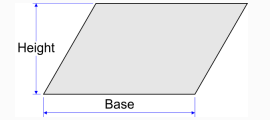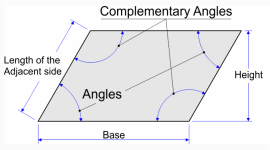# Calculating the Surface Area of a Parallelogram

The parallelogram has its parallel opposite sides that are the same length. The sum of its angles is equal to 360°. The degrees of each opposite angles are the same. The formula to determine the surface area is quite easy, since it's just base times height.

If you didn't happen to know the height, you can calculate the surface area by triangulation, using the quadrilateral module.

### Calculating the surface area of a parallelogram with base and height:

 Length of the base: Height: Unit: millimeter centimeter decimeter meter décameter hectometer kilometer inch foot yard mile Decimal places: 0 1 2 3 4 5 6 7 8 9 10
 Surface area: 2,00 m2## Calculating the surface area of a parallelogram with an angle and the length of the sides

 Length of the base: Length of one of the adjacent side: Unit: millimeter centimeter decimeter meter décameter hectometer kilometer inch foot yard mile One of the four Angles*: Degrees Radians Grades Decimal places: 0 1 2 3 4 5 6 7 8 9 10
 Surface area: 0,00 m2 Perimeter: 6,00 m Complementary angles*: 0,00 Height: 0,00 m## * Parallelogram angles

Enter the value of one of the four corners of the parallelogram, no matter which, the results remain the same in all scenarios. The value of complementary angles are by deduction. Complementary angles equals 180 minus the value of the initial angle.

For example, if you enter a narrow angle of 60 ° the complementary angles will be an open angle of 120 °. And vice versa, if you enter an open angle of 120 °, the complementary angles are an acute angle of 60 °. In both scenarios, the surface of the parallelogram remains the same, and its perimeter.